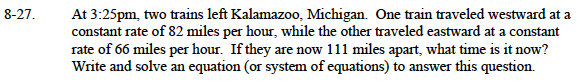### Home > CAAC > Chapter 8 > Lesson 8.1.3 > Problem8-27

8-27.Distance = (rate)(time)
Write a distance equation for each train.

If the trains are 111 miles apart, then their 'total distance' is 111. Use this to write an equation.

82t + 66t = 111
Note: The solution to this equation is not the answer to the question asked in the problem.